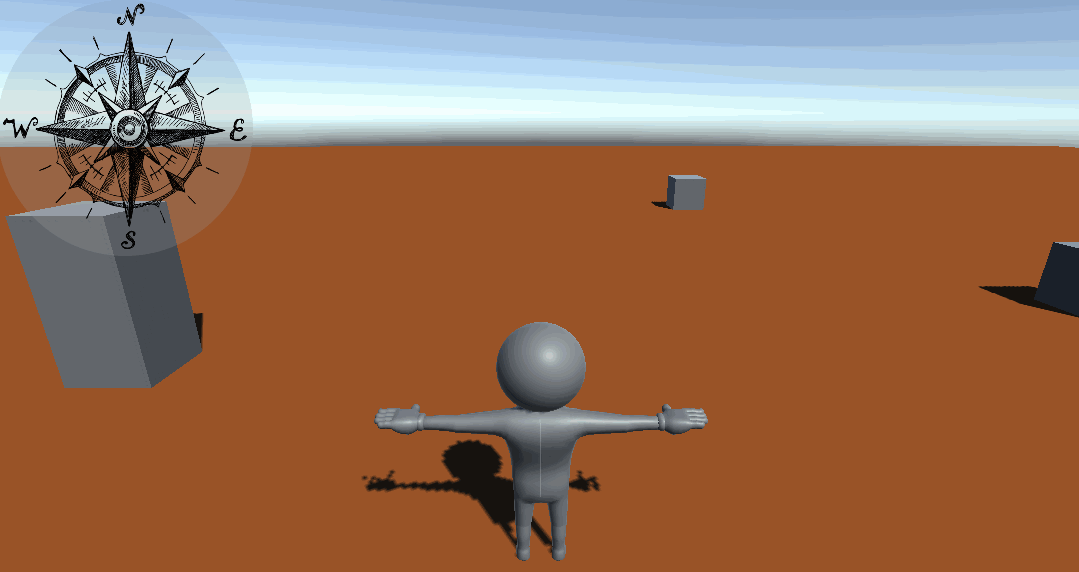When moving around in an expansive world in a game, there are 2 common solutions to help the player orientate: a minimap, or a compass.

In this article we’ll focus on implementing a compass, as it is simple and can feel much more authentic in a fantasy RPG than a full-blown minimap with objects and ennemies tracking.

You can also implement cool effects, such as making the Compass indicator spin like crazy to indicate treasure.

## From Player Orientation to Cardinal Direction

A compass is a fairly simple tool: wherever the player might be looking towards, it will always point North. To do this, we’ll create a canvas and an image with a compass texture. Then we’ll rotate the compass image on its z-axis, according to changes in the player’s orientation.

## Implementation

To do what we just described, we’ll create a Monobehaviour component that will transform the player’s forward into a rotation angle. If we consider North as being in the direction (0, 0, 1) in world space, then we can get the player’s forward, set the y value as 0, and normalize it.

By doing this, we can consider both points as being on a circle and use Mathf.Atan2 to get the angle by which we must rotate our sprite following the z-axis.

Then we’ll need to do a final adjustment: this angle calculation gives us the angle relative to the x-axis, so we need to add 90 degrees to set it relative to the z-axis.

This gives us the following code:

``````public class Compass : MonoBehaviour
{
public Transform mPlayerTransform;

[Tooltip("The direction towards which the compass points. Default for North is (0, 0, 1)")]
public Vector3 kReferenceVector = new Vector3(0, 0, 1);

// memalloc
private Vector3 _mTempVector;
private float _mTempAngle;

// Update is called once per frame
private void Update()
{
// get player transform, set y to 0 and normalize
_mTempVector = mPlayerTransform.forward;
_mTempVector.y = 0f;
_mTempVector = _mTempVector.normalized;

// get distance to reference, ensure y equals 0 and normalize
_mTempVector = _mTempVector - kReferenceVector;
_mTempVector.y = 0;
_mTempVector = _mTempVector.normalized;

// if the distance between the two vectors is 0, this causes an issue with angle computation afterwards
if (_mTempVector == Vector3.zero)
{
_mTempVector = new Vector3(1, 0, 0);
}

// compute the rotation angle in radians and adjust it
_mTempAngle = Mathf.Atan2(_mTempVector.x, _mTempVector.z);
_mTempAngle = (_mTempAngle * Mathf.Rad2Deg + 90f) * 2f;

// set rotation
transform.rotation = Quaternion.AngleAxis(_mTempAngle, kReferenceVector);

}
}
``````Congrats, our compass works as expected!